Next: Example of variance calculation Up: Annex: Variance of Sullivan Previous: Annex: Variance of Sullivan

## Estimate of variance of Sullivan index

It can be shown that the variances of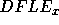and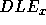can be approximated by: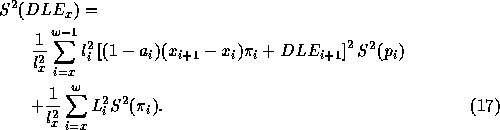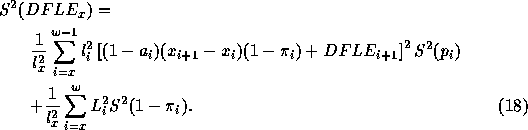whereand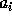be the mean of the fractions of the interval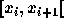lived by persons who die in the interval.

In case of life table use, the first term of equation 17: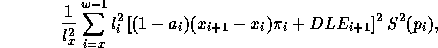is negligible in comparison with the other term (becauseis much smaller).

Generally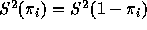can be approximated by: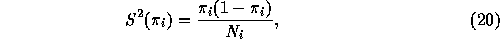where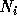is the number of persons of age i participating to the survey. So, equation 17 can be approximated by: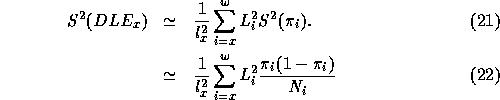And for the same reasons, equation 18 can also be approximated by: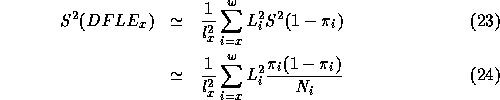Remark: In some cases, it could be more appropriate to take an other variance of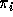than equation 20, related to the special characteristics of the sample used for the survey. In that case, reader needs to refer to the details of the survey itself (for example, in case of a stratified sample).

Eric Hauet
Fri Apr 25 22:40:35 DFT 1997